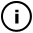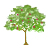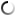# Sunday, July 04, 2010

## Yarn Galaxy (curls V5)

float x1old = 0,y1old = 0;
float x1current = 1,y1current = 0;
float x1new = 0,y1new = 0;
int counter = 0;
float curlSeed;
float rotation;
float wiggleSize = 1;

void setup() {
size(800,400);
background(255);
reStart();
drawVignettingEffect();
smooth();
}

void draw() {

smooth();
strokeWeight(1);
stroke(255.0*sin(counter/500.0),255.0*cos(counter/500.0),255.0*sin(counter/200.0),90-counter/200.0);
translate(width/2,height/2);
for(int i = 0;i < 200; i++){
counter++;
//x1 = 10;
//y1 = 0;
float s = counter/200.0;
rotation = (log(s/curlSeed))*tan(s);
//rotation = 0.1;

x1new = x1current + ((x1current-x1old) * cos(rotation) - (y1current-y1old) * sin(rotation)) ;
y1new = y1current + ((x1current-x1old) * sin(rotation) + (y1current-y1old) * cos(rotation));

point(x1new, y1new);

x1old = x1current;
x1current = x1new;
y1old = y1current;
y1current = y1new;

if (x1new > width/2) {
reStart();
return;
}

if (y1new > height/2) {
reStart();
return;
}

if (x1new < -width/2) {
reStart();
return;
}

if (y1new < -height/2) {
reStart();
return;
}
}
}

void reStart() {
x1old = 0;
y1old = 0;
float randomDirection = random(0,2*PI);
x1current = sin(randomDirection);
y1current = cos(randomDirection);
counter = 0;
curlSeed = random(5,20);

}

void drawVignettingEffect(){
smooth();
background(255);
strokeWeight(180);
noFill();
stroke(180,180,180,5);
translate(width/2,height/2);
for (int i = 0; i < 300; i+=10){
ellipse(0,0,max(width+i,height+i),max(width+i,height+i));
}
resetMatrix();
}

## infosubmitted by: DARYL_Gamma
views: 1496
There is a parallel dimension where, as Zaphod says, "everything appears to be made of yarn". This sketch represents a galaxy observed in such dimension, a galaxy made of yarn.This sketch has a parent
Tags: yarn, galaxy

## commentsloading...